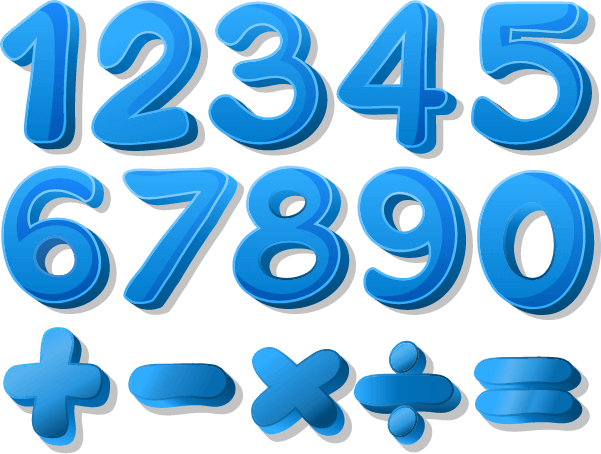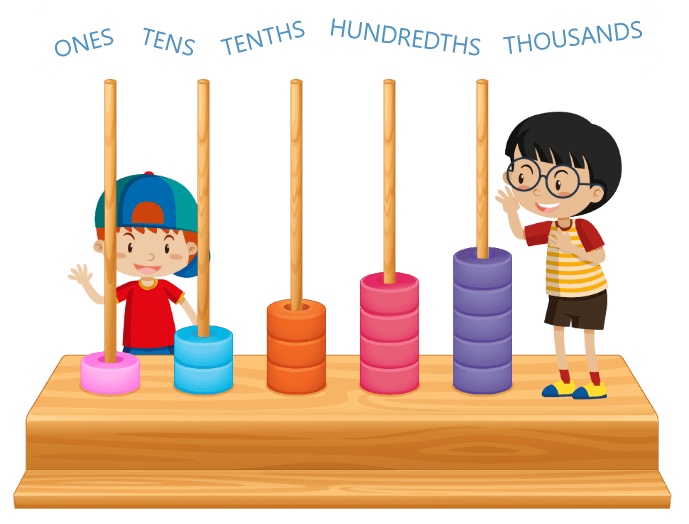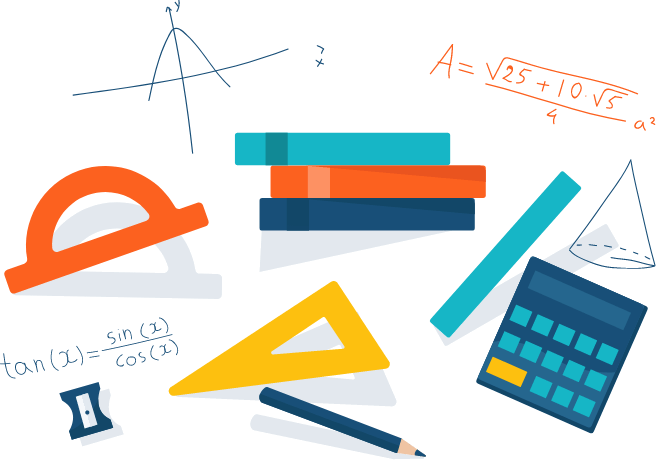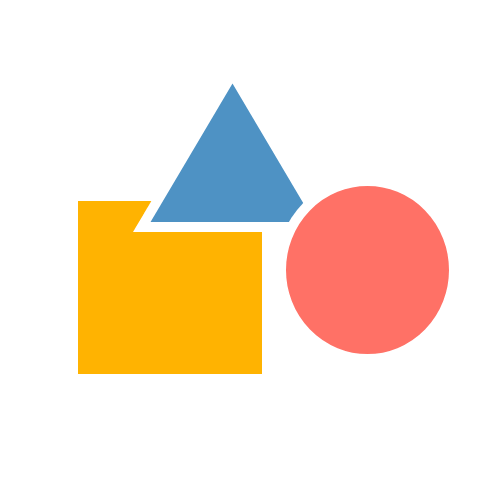# Math Grade - 1 Curriculum

Enter your email for the curriculum
A grade 1 child, at 98thPercentile, is exposed to four domains in mathematics as suggested by Common Core State Standards Initiative. The following is the snapshot of the skills which a child learns in grade 1.
Operations And Algebraic Thinking
Students develop strategies for adding andsubtracting whole numbers after learning numbers. They use a variety of models, including discrete objects and length-based models (e.g., cubes connected to for lengths), to model add-to, take-from, put-together, take-apart, comparesituations to understand the operations of addition and subtraction, and to develop strategies to solve arithmetic problems using these operations. Students understand connections among counting and, addition and subtraction (e.g., adding two is same as counting on two). By comparing a variety of solution strategies, children build their understanding of the relationship between addition and subtraction.Suggested Learning Goals For This Domain

After this domain, student will be able to:

• Represent and solve problems involving addition and subtraction.
• Understand and apply properties of operations and the relationship between addition and subtraction.
• Add and subtract within 20.
• Work with addition and subtraction equations.Numbers And Operations In Base Ten

Students develop, discuss, and use efficient, accurate, and generalized methods to add within 100 and subtract multiples of 10. They compare whole numbers (at least to 100) to develop understanding of and solve problems involving their relative sizes. They think of whole numbers between 10 and 100 in terms of tens and ones (especially recognizing the numbers 11 to 19 as composed of a ten and some ones).

Through activities that build number sense, they understand the order of the counting numbers and their relative magnitudes.

Suggested Learning Goals For This Domain

After this domain, student will be able to:

• Extend the counting sequence.
• Understand place value.
• Use place value understanding and properties of operations to add and subtract.
Measurement And Data
Students develop an understanding of the meaning and processes of measurement, including underlying concepts such as iterating (the mental activity of building up the length of an object with equal-sized units) and the transitivity principle for indirect measurement.Suggested Learning Goals For This Domain

After this domain, student will be able to:

• Measure lengths indirectly and by iterating length units.
• Tell and write time.
• Represent and interpret data.Geometry
Students compose and decompose plane or solid figures (e.g., put two triangles together to make a quadrilateral) and build understanding of part-whole relationships as well as the properties of the original and composite shapes. As they combine shapes, they recognize them from different perspectives and orientations, describe their geometric attributes, and determine how they are alike and different, to develop the background for measurement and for initial understandings of properties such as congruence and symmetry.
Suggested Learning Goals For This Domain

After this domain, student will be able to:

• Reason with shapes and their attributes.
• Tell and write time.
• Represent and interpret data.
Features of Math Grade 1 Program
• A Placement Test aimed at identifying grade-level proficiency in math
• 47 math tutoring sessions
• 5 Benchmark reviews conducted every month
• 4 Revision Classes covering entire syllabus
• An Exit Placement Test
• Final Benchmark Assessment to validate students’ grade-level proficiency

During the 47 tutoring sessions, a teacher ensures that student is prepared to meet the highest standards in math problem solving by supporting the child on daily basis through the following:

• Discussion on Critical Thinking Question
• Individual Skill Gap Analysis and Gap Filling
• Mandatory In-Class practice of concepts learned
• Minimum 30 minutes of daily practice through Homework
• Monthly celebrations based on the event theme merged with Math concepts

During this journey of students, at 98thPercentile, teachers ensure a regular interaction with parents. Parents stay connected through:

• Regular Parent-Teacher meeting aimed at sharing students’ progress
• A repository of recorded videos to help the child at home
• Printable lesson-wise worksheets to provide additional practice to the child
Disclaimer: The number of classes may increase or decrease depending on the discretion of the teacher. If a teacher reads the child has acquired grade specific skills she can decide to accelerate the child resulting in reduction in the number of classes, similarly when a child needs more support to acquire grade specific skills number of classes will go up.